Courses

WBJEE Chemistry Test - 5

40 Questions MCQ Test WBJEE Sample Papers, Section Wise & Full Mock Tests | WBJEE Chemistry Test - 5

Description
Attempt WBJEE Chemistry Test - 5 | 40 questions in 60 minutes | Mock test for JEE preparation | Free important questions MCQ to study WBJEE Sample Papers, Section Wise & Full Mock Tests for JEE Exam | Download free PDF with solutions
QUESTION: 1

Solution:
QUESTION: 2

Solution:
QUESTION: 3

Which of the following does not give red ppt. with Fehling solution?

Solution:
QUESTION: 4

The electronic configuration of metal M is 1s₂2s₂2p63s1. The formula of its oxide will be

Solution:
QUESTION: 5
A white crystalline substance dissolves in water. On passing H₂S in this solution, a black ppt. is obtained. The black ppt. dissolves completely in hot HNO₃. On adding a few drops of conc. H₂SO₄, a white ppt. is obtained. The ppt. is that of
Solution:
QUESTION: 6
Which of the following compound acts both nucleophile as well as an electrophile?
Solution:
QUESTION: 7
Enzyme trypsin converts
Solution:
QUESTION: 8

Which of the following ions has the maximum magnetic moment?

Solution:
QUESTION: 9

The enthalpy of formation for C2H4(g),CO2(g) and H2O(l) at 25ºC and 1 atm. pressure be 52,-394 and -286 kJ mol-1 respectively .The enthalpy of combustion of C2H4(g) will be

Solution:
QUESTION: 10

The equilibrium constant for the following reaction will be 3A + 2B → C

Solution:
QUESTION: 11
The half life of a reaction is halved as the initial conc. of the reactant is doubled. The order of the reaction is
Solution:
QUESTION: 12
The substance used in the preparation of malachine green is
Solution:
QUESTION: 13

The amount of heat required to raise the temperature of a body temperature through 1ºC is called, its

Solution:
QUESTION: 14
Which of the following exhibits geometrical isomerism ?
Solution:
QUESTION: 15
When AgNO₃ is strongly heated, the products formed are
Solution:
QUESTION: 16

In [Cr(C₂O₄)₃]3⁻, the isomerism shown is

Solution:
QUESTION: 17

Which of the following reacts with BCl3 to form diborane?

Solution:
QUESTION: 18
Electromagnetic separation is used in the concentration of
Solution:
QUESTION: 19
50 ml of gas A diffuses through a membrane in the same time as for the diffusion of 40 ml of a gas B under identical pressure-temperature conditions. If molecular weight of A is 64 that of B would be
Solution:
QUESTION: 20
Solution:
QUESTION: 21

Which of the following is correct about heavy water?

Solution:
QUESTION: 22
Total number of isomeric alcohols with formula C4H10O are :
Solution:
QUESTION: 23
Aniline reacts with KOH and phosgene to form
Solution:
QUESTION: 24
Isobar of a nucleide is formed when ...... takes place?
Solution:
QUESTION: 25
Which of the following is not a natural polymer?
Solution:
QUESTION: 26
Which of the following is formed by polymerisation of styrene in the presence of benzoyl peroxide?
Solution:
QUESTION: 27
Oxidation state of +1 for phosphorus is found in
Solution:
QUESTION: 28
Ionic solids with Schottky defects contain in their structure
Solution:
QUESTION: 29
The prefix D-in the notation for specific rotation of an optically active compound refers to :
Solution:
QUESTION: 30
The correct order of reducing abilities of hydrides of V group elements is
Solution:
QUESTION: 31

In the following question, a Statement of Assertion (A) is given followed by a corresponding Reason (R) just below it. Read the Statements carefully and mark the correct answer-
Assertion(A):Ether and alcohol are water soluble
Reason(R):Both Ether and alcohol can form stable H-bonds with H2O molecules.

Solution:
QUESTION: 32

Which one of the following pairs of species have the same bond order?

Solution:

Bond order of CN = Bond order of NO+ = 3.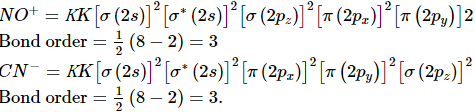QUESTION: 33

Alkene R-CH=CH₂ reacts readily with B₂H₆ and the product on oxidation with alkaline hydrogen perioxide produces

Solution:
QUESTION: 34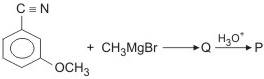The product 'P in the above reaction is

Solution:

The reaction proceeds as :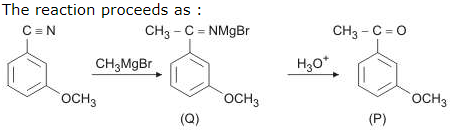QUESTION: 35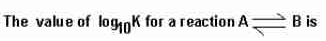(Given : ∆r298K=-54.07kJ mol⁻1, ∆r298K=10JK⁻1mol⁻1 and R= 8.314 JK⁻1mol⁻1; 2.303x8.314x298 = 5705)

Solution:
*Multiple options can be correct
QUESTION: 36

Which of the following compounds exhibit optical activity?

Solution:
*Multiple options can be correct
QUESTION: 37

In electrolysis of very dilute NaOH solution using platinum electrodes

Solution:
*Multiple options can be correct
QUESTION: 38

The correct statement(s) about the compound given below is (are)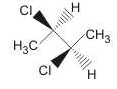Solution:
*Multiple options can be correct
QUESTION: 39

Formic acid gives the test of:

Solution:
*Multiple options can be correct
QUESTION: 40

Which statement(s) about cathode rays is/are correct?

Solution:Use Code STAYHOME200 and get INR 200 additional OFF Use Coupon Code

Track your progress, build streaks, highlight & save important lessons and more!

Similar ContentRelated tests